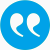#Pötzelberger, Klaus. 2021. On the empirical estimator of the boundary in inverse first-exit problems. Computational Statistics. 36 (1), 1809-1820.

BibTeX

## Abstract

First-exit problems for the Brownian motion (W(t)) or general diffusion processes, have important applications. Given a boundary b(t), the distribution of the first-exit time τ has to be computed, in most cases numerically. In the inverse first-passagetime problems, the distribution of τ is given and the boundary b has to be found. The boundary and the density of τ satisfy a Volterra integral equation. Again numerical methods approximate the solution b for given distribution of τ. We propose and analyze estimators of b for a given sample τ1, . . . , τn of first-exit times. The first estimator, the empirical estimator, is the solution of a stochastic version of the Volterra equation. We prove that it is strongly consistent and we derive an upper bound for its asymptotics convergence rate. Finally, this estimator is compared to a Bayesian estimator, which is based on an approximate likelihood function. Monte Carlo experiments suggests that the empirical estimator is simple, computationally manageable and outperforms the alternative procedure considered in this paper.

## Tags

Press 'enter' for creating the tag

## Publication's profile

Status of publication Published WU Journal article Computational Statistics SCI FIN-A English On the empirical estimator of the boundary in inverse first-exit problems 36 1 2021 1809 1820 Y https://link.springer.com/article/10.1007/s00180-020-00989-x http//dx.doi.org/10.1007/s00180-020-00989-x N C13, C14

## Associations

People
Pötzelberger, Klaus (Details)
Organization
Institute for Statistics and Mathematics IN (Details)
Research areas (ÖSTAT Classification 'Statistik Austria')
1104 Applied mathematics (Details)
1113 Mathematical statistics (Details)
1118 Probability theory (Details)